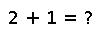# eachindex

eachindex(A...)

Creates an iterable object for visiting each index of an AbstractArray `A` in an efficient manner. For array types that have opted into fast linear indexing (like `Array`), this is simply the range `1:length(A)`. For other array types, this returns a specialized Cartesian range to efficiently index into the array with indices specified for every dimension. For other iterables, including strings and dictionaries, this returns an iterator object supporting arbitrary index types (e.g. unevenly spaced or non-integer indices).

Example for a sparse 2-d array:

``````julia> A = sprand(2, 3, 0.5)
2x3 sparse matrix with 4 Float64 entries:
[1, 1]  =  0.598888
[1, 2]  =  0.0230247
[1, 3]  =  0.486499
[2, 3]  =  0.809041

julia> for iter in eachindex(A)
@show iter.I_1, iter.I_2
@show A[iter]
end
(iter.I_1,iter.I_2) = (1,1)
A[iter] = 0.5988881393454597
(iter.I_1,iter.I_2) = (2,1)
A[iter] = 0.0
(iter.I_1,iter.I_2) = (1,2)
A[iter] = 0.02302469881746183
(iter.I_1,iter.I_2) = (2,2)
A[iter] = 0.0
(iter.I_1,iter.I_2) = (1,3)
A[iter] = 0.4864987874354343
(iter.I_1,iter.I_2) = (2,3)
A[iter] = 0.8090413606455655``````

If you supply more than one `AbstractArray` argument, `eachindex` will create an iterable object that is fast for all arguments (a `UnitRange` if all inputs have fast linear indexing, a CartesianRange otherwise). If the arrays have different sizes and/or dimensionalities, `eachindex` returns an iterable that spans the largest range along each dimension.

## Examples

Checking you are not a robot: